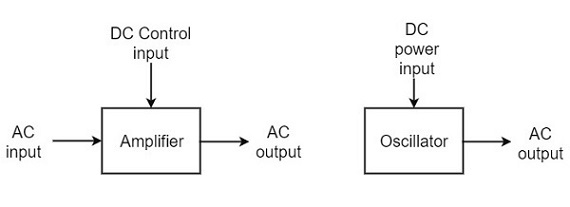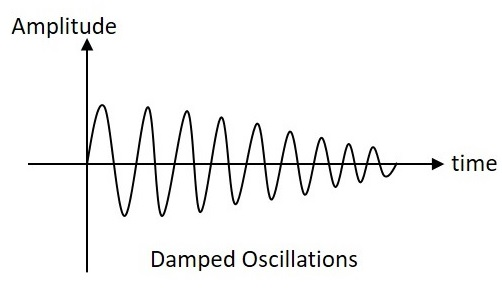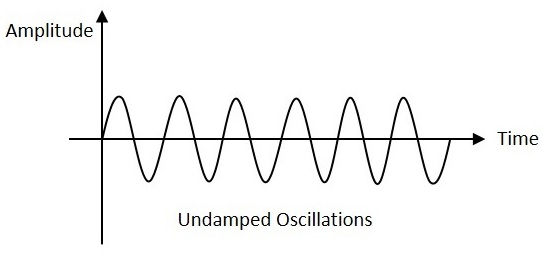# Sinusoidal Oscillators - Introduction

An oscillator generates output without any ac input signal. An electronic oscillator is a circuit which converts dc energy into ac at a very high frequency. An amplifier with a positive feedback can be understood as an oscillator.

### Amplifier vs. Oscillator

An amplifier increases the signal strength of the input signal applied, whereas an oscillator generates a signal without that input signal, but it requires dc for its operation. This is the main difference between an amplifier and an oscillator.

Take a look at the following illustration. It clearly shows how an amplifier takes energy from d.c. power source and converts it into a.c. energy at signal frequency. An oscillator produces an oscillating a.c. signal on its own.The frequency, waveform, and magnitude of a.c. power generated by an amplifier, is controlled by the a.c. signal voltage applied at the input, whereas those for an oscillator are controlled by the components in the circuit itself, which means no external controlling voltage is required.

### Alternator vs. Oscillator

An alternator is a mechanical device that produces sinusoidal waves without any input. This a.c. generating machine is used to generate frequencies up to 1000Hz. The output frequency depends on the number of poles and the speed of rotation of the armature.

The following points highlight the differences between an alternator and an oscillator −

• An alternator converts mechanical energy to a.c. energy, whereas the oscillator converts d.c. energy into a.c. energy.

• An oscillator can produce higher frequencies of several MHz whereas an alternator cannot.

• An alternator has rotating parts, whereas an electronic oscillator doesn’t.

• It is easy to change the frequency of oscillations in an oscillator than in an alternator.

Oscillators can also be considered as opposite to rectifiers that convert a.c. to d.c. as these convert d.c. to a.c. You can get a detailed description on rectifiers in our Electronic Circuits tutorial.

## Classification of Oscillators

Electronic oscillators are classified mainly into the following two categories −

• Sinusoidal Oscillators − The oscillators that produce an output having a sine waveform are called sinusoidal or harmonic oscillators. Such oscillators can provide output at frequencies ranging from 20 Hz to 1 GHz.

• Non-sinusoidal Oscillators − The oscillators that produce an output having a square, rectangular or saw-tooth waveform are called non-sinusoidal or relaxation oscillators. Such oscillators can provide output at frequencies ranging from 0 Hz to 20 MHz.

We will discuss only about Sinusoidal Oscillators in this tutorial. You can learn the functions of non-sinusoidal oscillators from our Pulse Circuits tutorial.

## Sinusoidal Oscillators

Sinusoidal oscillators can be classified in the following categories −

• Tuned Circuit Oscillators − These oscillators use a tuned-circuit consisting of inductors (L) and capacitors (C) and are used to generate high-frequency signals. Thus they are also known as radio frequency R.F. oscillators. Such oscillators are Hartley, Colpitts, Clapp-oscillators etc.

• RC Oscillators − There oscillators use resistors and capacitors and are used to generate low or audio-frequency signals. Thus they are also known as audio-frequency (A.F.) oscillators. Such oscillators are Phase –shift and Wein-bridge oscillators.

• Crystal Oscillators − These oscillators use quartz crystals and are used to generate highly stabilized output signal with frequencies up to 10 MHz. The Piezo oscillator is an example of a crystal oscillator.

• Negative-resistance Oscillator − These oscillators use negative-resistance characteristic of the devices such as tunnel devices. A tuned diode oscillator is an example of a negative-resistance oscillator.

## Nature of Sinusoidal Oscillations

The nature of oscillations in a sinusoidal wave are generally of two types. They are damped and undamped oscillations.

### Damped Oscillations

The electrical oscillations whose amplitude goes on decreasing with time are called as Damped Oscillations. The frequency of the damped oscillations may remain constant depending upon the circuit parameters.Damped oscillations are generally produced by the oscillatory circuits that produce power losses and doesn’t compensate if required.

### Undamped Oscillations

The electrical oscillations whose amplitude remains constant with time are called as Undamped Oscillations. The frequency of the Undamped oscillations remains constant.Undamped oscillations are generally produced by the oscillatory circuits that produce no power losses and follow compensation techniques if any power losses occur.